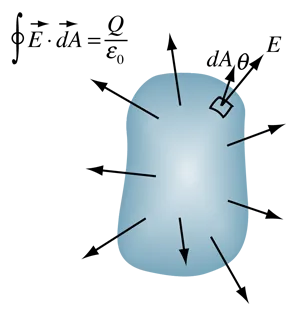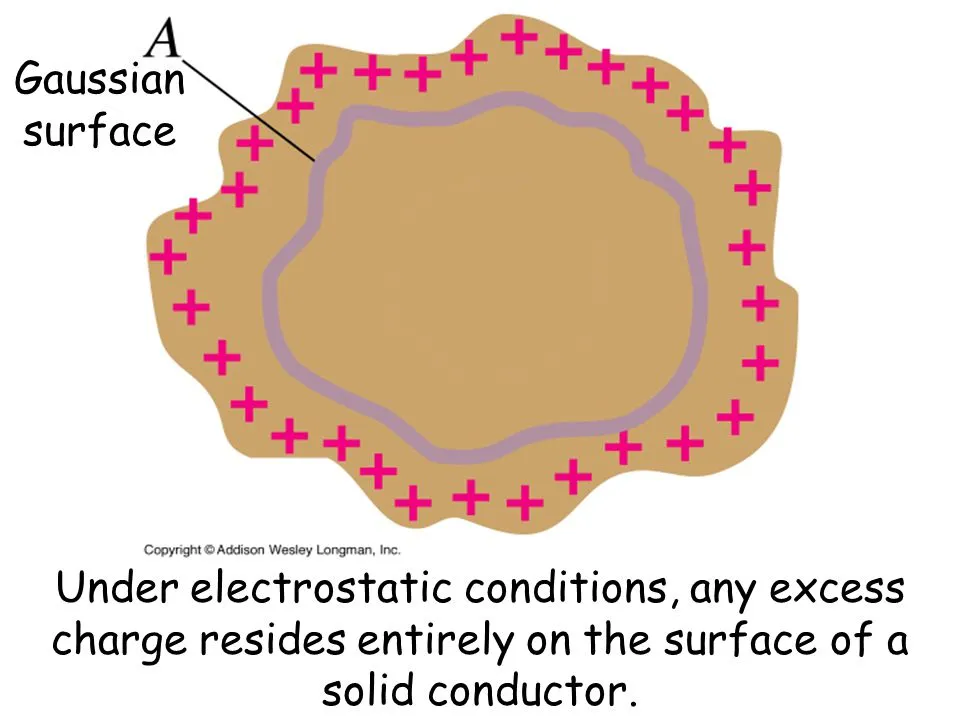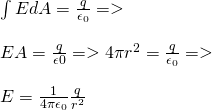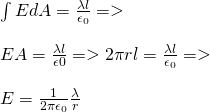# Physics - Electromagnetism - Applications of Gauss's law (part 1)Source

## Introduction

Hello it's a me again Drifter Programming!

Today's topic of Physics in the branch of Electromagnetism is again Gauss's law! This time we will cover the Applications of this law and not the "basic theory" behind it. I will split this post in parts and actual "mathematical" examples will come in an upcoming post :)

So, without further do, let's get started!

## Applying Gauss's law

First of all, Gauss's law applies to any distribution of charge and for any surface.

We can use the equation in mainly two ways:

• When knowing an distribution of charge with enough symmetry then we can calculate the integral of Gauss's law and so can calculate the electric field.
• When knowing the electric field we can use the law to find the distribution of charge, such as charges on top of conductive surfaces.

Some critical points/tips when applying the law:

1. The first step when applying Gauss's law is of course the selection of the so called Gaussian surface (or G.S. in short) that we are going to use with Gauss's law. When calculating the electric feild at some specific point we of course have to make sure that the point is on top of that Gaussian surface.
2. The Gaussian surface doesn't have to be a real surface (as I already pointed out last time). Often the appropriate geometrical surface is an imaginery surface.
3. The Gaussian surface and the distribution of charge need to have some common property of symmetry, so that it's possible to calculate the integral. So, when the distribution of charge is an sphere then the G. S. will also be an concentric sphere.
4. We often split an closed G. S. in to many separate surfaces. Of course the integral of the total closed surface is equal to the sum of the integral of each separate surface (some could also equal zero).
5. When an electric field E is vertically across at some point of a surface with area A and also has the same meter at each point of the surface, then the electric field is constant and so the integral of the total surface is equal to EA.
6. When an electric field E is tangent at every point of a surface, then the Gauss integral on top of the whole surface is zero.
7. When E = 0 at every point of a surface, then the integral is zero.
8. Generally, the electric field is partially caused by charges inside of the volume and partially caused by charged outside of the volume of the surface. This means that the electric field at points inside of the surface is not necessarily zero, even when there are no charges inside of that surface. But, at this last case the integral of that closed surface is of course always zero.

## Gauss Applications

Let's now get into some Applications for simple distributions of charge...

### Locating excess charge at a solid conductor

When excess charge is placed at a solid conductor and when the stability has been restored, then the charge resides throughout the surface and not in the internal of the conductor.

Let's proof this...

We know from Electrostatics (charge at rest) that the electric field E at every point inside of an conductive material is zero, cause if not then the charges would move and so the material would be unstable.

Suppose that we "create" an Gaussian surface with area A in the inside of the conductor. Because E = 0 at every point of the entire surface we know from Gauss's law that the total charge inside is also zero.

Let's shrink this surface until we can consider the surface a point. Of course this point charge must have charge zero. We can apply this for any point inside of the conductor and so there can't be any point with charge inside of the conductor.

So, any excess charge (at static condition or stability) on top of a solid conductor is distributed along the surface like that:Source

This was more of an theoretical application, but I think that it helps you understand what it means to have no electric field (E = 0) in the inside of a conductor.

### Electric field of a charged conductive sphere

Suppose we have a solid, charged, conductive sphere of charge q and radius R.

What is the meter of the electric field at any point outside of that sphere?

Using the previous application's knowledge we can say that all the charge resides on the surface of the sphere. The sphere is of course symmetrical and so has the charge uniformly distributed throughout the surface. This means that the electric field's direction at any point P outside of the sphere is the radial line between the center of the sphere and the point P.

The electric field is of course radial and so the meter of E at all the points of a spherical surface with radius r that is concentric to the solid sphere is equal.

Let's suppose such an Gaussian surface which is a sphere with radius r, which is bigger then the radius R of the conductive sphere. The area of that surface is of course 4πr^2.

Because the electric field is vertically across at every point we have:quicklatex

This equation is exactly the same that we had with point charges!

This means that the electric field that is caused by the sphere is equal to the one that would be caused if the charge was concentrated at the center of the sphere.

Exactly at the surface of the sphere where r = R we have:quicklatex

When r < R the electric field is of course zero and so E = 0.

The limit as R -> 0 gives us Coulomb's law!

Something worth noting is that we get the same results if we replace the sphere with a conductive cortex sphere.

### Linear electric fields

Electric charge is distributed evenly/uniformly along a very long wire.

The charge per unit length is λ.

What is the electric field?

Well, there again is a symmetry, because we can rotate and move the system in any axis and nothing really changes. Using the first argument again we conclude that the electric field at each point doesn't change by applying rotations or by moving the system.

There also cannot be an component of the electric field that is parallel to the wire, cause else we would have to separate one end of the other in some way, which we don't have.

In addition to that there can't be an component of the electric field that is tangent to a circle that is vertically across to the wire, cause else we would again have to explain in which direction it goes.

So, what remains now are the radial components. That way, because the wire is very long, the electric field lines at any point that is not at the ends of the wire are in planes that are vertically across to the wire. The meter of the electric field of course only depends on the radial distance with regard to the wire.

Let's suppose an Gaussian surface which is an cylinder of radius r and arbitrary length l with the bases vertically across to the wire. The total enclosed charge is Qencl = λl.

By analyzing the surface integral into parts which are one for each base and one for the side, we have that the electric field E and elementary areas dA at the bases are vertically across and so don't contribute to the integral. This means that the only thing contributing is the side of the cylinder.

For symmetry reasons, the electric field E is vertically across to the side and parallel to dA at each point of it and so the electric field E is equal to the vertically across component of E and equal at any point of the side surface.

The area of that surface is A = 2πrl and so we end up with:quicklatex

You can see that the length of the cylinder doens't play a role anymore!

I would like to note that we would get to the same conlusion if not the entire charge was inside of the cylinder. The "equation" also works for wires with small length, but when getting to actual points Coulomb's law should be prefered, compared to Gauss's law.

The same equation also works if we had an charged cylinder (that could even be a cortex) instead of a wire, cause the conclusion will be the same as when having the charge concentrated in the central axis of the cylinder.

Intro

Physics Introduction -> what is physics?, Models, Measuring

Vector Math and Operations -> Vector mathematics and operations (actually mathematical analysis, but I don't got into that before-hand :P)

Classical Mechanics

Velocity and acceleration in a rectlinear motion -> velocity, accelaration and averages of those

Rectlinear motion with constant accelaration and free falling -> const accelaration motion and free fall

Rectlinear motion with variable acceleration and velocity relativity -> integrations to calculate pos and velocity, relative velocity

Rectlinear motion exercises -> examples and tasks in rectlinear motion

Position, velocity and acceleration vectors in a plane motion -> position, velocity and accelaration in plane motion

Projectile motion as a plane motion -> missile/bullet motion as a plane motion

Smooth Circular motion -> smooth circular motion theory

Plane motion exercises -> examples and tasks in plane motions

Force and Newton's first law -> force, 1st law

Mass and Newton's second law -> mass, 2nd law

Newton's 3rd law and mass vs weight -> mass vs weight, 3rd law, friction

Applying Newton's Laws -> free-body diagram, point equilibrium and 2nd law applications

Contact forces and friction -> contact force, friction

Dynamics of Circular motion -> circular motion dynamics, applications

Object equilibrium and 2nd law application examples -> examples of object equilibrium and 2nd law applications

Contact force and friction examples -> exercises in force and friction

Circular dynamic and vertical circle motion examples -> exercises in circular dynamics

Electromagnetism

Getting into Electromagnetism -> electromagnetim, electric charge, conductors, insulators, quantization

Coulomb's law with examples -> Coulomb's law, superposition principle, Coulomb constant, how to solve problems, examples

Electric fields and field lines -> Electric fields, Solving problems around Electric fields and field lines

Electric dipoles -> Electric dipole, torque, potential and field

Electric charge and field Exercises -> examples in electric charges and fields

Electric flux and Gauss's law -> Electric flux, Gauss's law

And this is actually it for today and I hope that you enjoyed it!

Next time we will get into part 2!

Bye!

H2
H3
H4
3 columns
2 columns
1 column
1 Comment